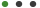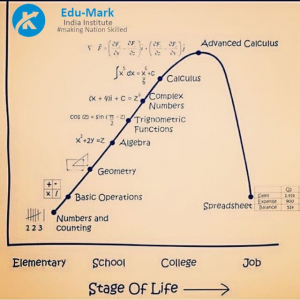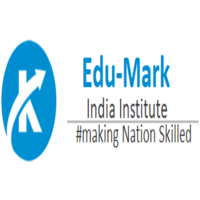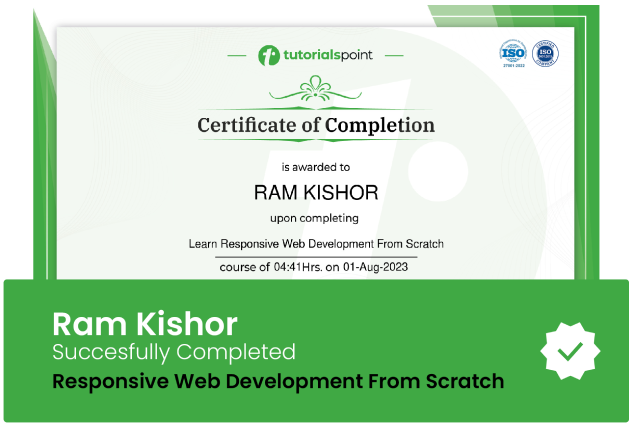### Advanced Mathematics Preparation for JEE/CET/CATEdu - Mark India

# Advanced Mathematics Preparation for JEE/CET/CAT

Learn and become master in Advance MathematicsUpdated on Sep, 2023Language - EnglishEdu - Mark IndiaTeaching & Academics,Math,Algebra30-days Money-Back Guarantee

Training 5 or more people ?

# Mathematics is considered as one of the challenging subjects, therefore, we have designed this course by keeping in mind all the needs of students.

1.  Mathematical Logic
2. Matrics
3. Trigonometric function
4. Pair of Straight line
5. Linear Programming
6. Line and Plane

### :::: Who are your target students? :::::

• Mathematician facing problems in Algebra and Advance mathematics likes ALGEBRA & GEOMETRY
• Beginner Class for Mathematics and Advance ALGEBRA & GEOMETRY
• A beginner who is curious for mathematics like Linear Programming, Logic, Matrices, Trigonometry, Geometry Theorems
• Homeschool parents looking for extra support with Mathematic
• Anyone who wants to study advanced mathematics, ALGEBRA & GEOMETRYfor fun after being away from school for a while

### ::: What will you Learn in THIS COURSE:::

• Mathematical Logics with statements and Sentences
• Matrices and their Basic Operation and types
• Trigonometric Function and Problems regarding Trigonometry
• Problems related to pair of Straight Line
• Lines & Plane
• Linear Programming (LP) and how to study them and future use of it

### :::: What ARE the benefits for this COURSE:::::

• On completion of this course, one will have detailed knowledge of all the chapters and can easily solve all the problems which can lead to score well in exams with the help of:
• Explanatory videos ensure complete concept understanding.

• Quiz Video helps in testing your knowledge

• In short, it is an interesting course fulfilling all the student's needs.

• Certificate after Accomplishing the course

• QUARTERLY(3months) UPDATES ON THIS COURSE

### Goals

What will you learn in this course:

• Mathematical Logics with statements and Sentences
• Matrices and their Basic Operation and types
• Trigonometric Function and Problems regarding Trigonometry
• Problems related to pair of Straight Line
• Vector Operations , Using vector understand the logic and need of it
• Lines & Planes
• Linear Programming (LP) and how to study them and future use of it

### Prerequisites

What are the prerequisites for this course?

• You should be comfortable with arithmetic (addition, subtraction, multiplication, division) of whole numbers.
• You should be comfortable with arithmetic of decimals, fractions, exponents, and radicals.
• We'll start the course with basic understanding and knowledge of algebraic operations, so if you know those arithmetic basics, you'll be well prepared for the course.## Curriculum

Check out the detailed breakdown of what’s inside the course

Introduction to course
1 Lectures
•Introduction 00:31 00:31
Mathematical LOGIC
7 LecturesMathematical Matrices
3 LecturesTrigonometric Functions
5 LecturesPair of Straight Line
3 LecturesLines & Plane
8 LecturesLinear Programming
3 Lectures## Instructor DetailsEdu - Mark India

An Educational Markerting Company

## Course Certificate

User your certification to make a career change or to advance in your current career. Salaries are among the highest in the world.